﻿ Calculus Students’ Visual Thinking of Definite Integral

### Calculus Students’ Visual Thinking of Definite Integral

Chih Hsien HuangOPEN ACCESSPEER-REVIEWED

## Calculus Students’ Visual Thinking of Definite Integral

Chih Hsien HuangDepartment of Electrical Engineering, Ming Chi University of Technology, New Taipei City, Taiwan, ROC

### Abstract

Visualization as both the product and the process of creation, interpretation and reflection upon pictures and images, is gaining increased visibility in mathematics and mathematics education. The use of diagrams to visualize definite integral concept, however, is problematic for many students and may actually hinder their problem-solving efforts. The purpose of this study, not only extends our understanding of students’ difficulties and strengths associated with visualization but also identifies types of visual image they utilized while solve integral problems. Through the detailed analyses of students' work and verbal protocols, the students with high visualization ability use of imagination images in high percentages along with algebraic representations and linking these two representations lead to the success of problem solving. The students with low visualization ability use of memory images. It is discovered that students can produce imagination images that play a significant role in a problem solving process. As such, a process of visualization allows an articulation between representations to produce another representation that could help students solve given problems.

### At a glance: Figures

123
Prev Next

• Huang, Chih Hsien. "Calculus Students’ Visual Thinking of Definite Integral." American Journal of Educational Research 3.4 (2015): 476-482.
• Huang, C. H. (2015). Calculus Students’ Visual Thinking of Definite Integral. American Journal of Educational Research, 3(4), 476-482.
• Huang, Chih Hsien. "Calculus Students’ Visual Thinking of Definite Integral." American Journal of Educational Research 3, no. 4 (2015): 476-482.

 Import into BibTeX Import into EndNote Import into RefMan Import into RefWorks

### 1. Introduction

The research literature on mathematics education has long discussed the merits of visualization and analysis in mathematical thinking. Visualization has been an area of interest for a number of researchers concerned with mathematics education. Many researchers emphasize the importance of visualization and visual reasoning for learning mathematics, and visualization is a fundamental aspect to understand students’ construction of mathematical concepts [1, 5, 21, 26]. In their arguments for visualization, suggest that visual thinking can be an alternative and powerful resource for students doing mathematics, a resource that can open the way to different ways of thinking about mathematics than the linguistic and logico-propositional thinking of traditional proofs and the symbol manipulation of traditional algebra. To use this template, you will need to (1) apply the embedded styles to each paragraph-level item in your manuscript or (2) use the specifications shown in Table 1 to format your manuscript, with this template as a visual guide. Information about paper submission is available from the Journal website.

According to Zimmerman , “the role of visual thinking is so fundamental to the understanding of calculus that is difficult to imagine a successful calculus course which does not emphasize the visual elements of the subject”. Although visualization must necessarily play a important role in mathematical activity, it clearly need research which helps us understand more about which of their features contribute significantly to the role in a given mathematical situation. There have many studies focus on the derivative and antiderivative graphs [10, 23], but there is few research focus on the concept of definite integral. This study stands apart from other research on learning calculus because it not only extends our understanding of students’ difficulties and strengths associated with visualization but also identifies types of visual image they utilized while solve integral problems. This study addressed the following research questions: What aspects of the use of different types of imagery and visualization are effective in definite integral problem solving? What visual or analytic mental processes do calculus students use to understand the concept of definite integral?

### 2. Literature Review

2.1. Representations of Concepts

Kaput  addresses the importance of theoretical framework of representation systems because of the difficulties students experience in translating between different representations of mathematical concepts. The students of the future will be choosing how to represent given relationships and the skill of constructing or choosing relationships will be more important than computational skills. From a constructivist point of view, “perceiving” is an active process and not a passive one because “the representation does not represent by itself. It needs interpreting and to be interpreted, it needs an interpreter” . The students’ interpretation resides in their activities not in the objects the students perceive. The students’ concepts, mental representations, and images are dynamic and are not replicas of external world.

Representation is an indispensable tool for presenting mathematical concepts, communicating and considering or thinking. Hiebert and Carpenter  assessed students’ understanding of concepts based on the relationships between the representations they created. They contended that “the mathematics is understood if its mental representation is part of a network of representations. The degree of understanding is determined by the number and the strength of the connections (p. 67)”. This perspective supports that proposed by Duval . Duval maintained that the process of mathematical thinking required not only the use of representation systems (which Duval called registers) but also cognitive integration of representation systems. Based on Duval’s analysis, learning and comprehending mathematics require relatively similar semiotic representations. From this perspective, the understanding of a mathematical concept is built through tasks that imply the use of different systems of representation and promote the flexible coordination between representations. Therefore, learning mathematics implies “the construction of a cognitive structure by which the students can recognize the same object through different representations” .

The learning of calculus movement emphasizes the use of multiple representations in the presentation of concepts—that concepts should be represented numerically, algebraically, graphically, and verbally wherever possible—so that students understand connections between different representations and develop deeper and more robust understanding of the concepts [10, 13].

The essence of the concept of integrals (including other mathematical concepts as well) is that the process concept and object concept can be presented by connected but different representations. A number of studies have indicated that the representations used by students to solve an integral problem are related to the meanings they attribute to the concept of integrals . The graphical representation of definite integrals is typically used in calculations that involve areas under a curve, whereas numerical representations are used for Riemann’s cumulative addition problems . Solving integrals using common integration techniques demonstrates the need for symbolic representations.

In this study, we situated our investigation of representation theory within the context of integrals. Specifically, we examined students’ ability to use the relationship between representations to solve integral problems. In our specific case - the understanding of the concept of integral- research conducted with this representational approach highlights as a cause of these difficulties the lack of coordination between both the graphic and algebraic representations and the predominance of the latter in the students’ answers. This leads us to pay special attention to the use of the graphical representation and to visualization.

2.2. Visualization in Mathematics Learning

Visualization is a critical aspect of mathematical thinking, understanding, and reasoning. Researchers argue that visual thinking is an alternative and powerful resource for students to do mathematics, it is different from linguistic, logico-propositional thinking and manipulation of symbols. A growing body of research supports the assertion that understanding of mathematics is strongly related to the ability to use visual and analytic thinking. Researchers contend that in order for students to construct a rich understanding of mathematical concepts, both visual and analytic reasoning must be present and integrated [2, 24].

According to Duval , visualization can be produced in any register of representation as it refers to processes linked to the visual perception and then to vision. Zimmerman and Cunningham  contended that the use of the term “visualization” concerned a concept or problem involving visualizing. Nemirovsky and Noble  defined visualization as a tool that penetrated or travelled back and forth between external representations and learners’ mental perceptions. Goldin  and Hitt  both emphasized the relationships among representation, mathematical visualization, and conceptual understanding. Dreyfus contended that what students “see” in a representation would be linked to their conceptual structure, and further proposed that visualization should be regarded as a learning tool. Noss, Healy, and Hoyles  described mathematical thinking as being characterized by the ability to move freely between the visual and the symbolic, the formal and the informal, the analytical and the perceptual, and the rigorous and the intuitive.

Visualization involves both external and internal representations (or images), and thus following Presmeg , we define visualization as processes involved in constructing and transforming both visual images and all of the representations of a spatial nature that may be used in drawing figures or constructing or manipulating them with pencil and paper. This definition emphasizes that in mathematical thinking and problem solving, an appropriate graph can be drawn to represent the mathematical concept or question, and that the graph can be used to understand a concept or as a problem-solving tool. In this study, we investigated the visual images that students used to resolve specific problems and how they managed given visualizations.

### 3. Method

3.1. Participants and Instruments

The 15 first-year engineering students who participated in this study were enrolled at a university of technology and had learned the basic rules of integration using primitives, as well as their relationship to the calculation of a number of areas under curves. These students’ calculus grades in the top 10% among 352 students. The instruments used for data collection were a questionnaire containing problems and interviews. The questionnaire comprised five problems in definite integral (Figure 1), some of which were referenced from other studies [7, 15]. These problems enabled the students’ performance regarding the visual thinking to be analyzed. The results of the questionnaire necessitated further investigation into the visual thinking of students. The clinical interviews were carried out after the answers to the problems had been analyzed . Each interview lasted about 40-50 minutes and was video- and audio-taped. In order to prepare the script for the interview, we analyzed the written answers focused on in relation to how the students seemed to use and coordinate the different mathematical representations needed. During each interview and were asked to think aloud while they were solving the tasks so that we could describe their responses and strategies as well as make inferences about their mental processes and images.

3.2. Analysis

The analysis focused on identifying the students’ mental processes and images used to create meanings for the problems and the justifications provided. For each problem, we identified:

-The visual images and representations that the student used.

-The relationships that the student established between the visual images and representations to generate new information.

This analysis provides information on how the student uses and relates the representations of mathematical knowledge to obtain new information.

According to these data analyses, to assess and interpret the visual thinking of the definite integral concept of students in this study, we constructed the visual thinking structure of definite integrals, employed this structure to develop clear standards, and then classify the visual thinking of the students into various levels. The standards were related to the thinking process adopted and presented by the students when problem solving, as well as their potential to construct relationships among the various representations and properties, and the degree to which they integrated these relationships into their explanation of problem solving. The analysis results indicated that the visual thinking distribution of 15 students could be categorized to 5 competencies and three levels. In the non-visual (NV) level, one tends to focus on a single visual image, overlooking other representations of a similar nature. For example, students believed that they had to perform integral operations to determine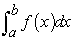. In the local-visual (LV) level, one can perceive and confirm the relationships among various visual images; however, these items may still appear independent of each other. For example, students understood the relationship between the integral and area, but were unable to differentiate between the relationships of areas above and below the x axis with the integral. In the global-visual (GV) level, one can use the relationships to construct a consistent structure based on the relationships among various visual images. The importance of this consistency lies in the fact that it determines the scope of visual thinking.

### 4. Result and Discussion

According to the data analysis, we identify 5 competencies of visual thinking regarding to the concept of definite integral, and then classify the visual thinking of the students into three levels (Table 1). Because students’ visual thinking of the definite integral could be reasonably understood regarding the three levels, we evaluated the responses to the task interviews, searching for evidence of the NV, LV, and GV levels. Most students appeared to be in either the NV or LV levels, and the difference was usually obvious, as demonstrated by the representative examples included in the following discussion.

#### Table 1. Visual thinking abilities of three levelsDownload asPowerPoint Slide

Veiw figureView current table in a new window

4.1. Visual Thinking of Definite Integral at the NV Level

The initial level of visual thinking is the non-visual level. We categorized four students into this group. One of the visual thinking characteristics shared by these students was that they could not recognize the relationship between the area and integral. These students could only process representations within a representation system, and the representations used were influenced by the representation format employed for problems. Additionally, they preferred solving problems using symbolic representations.

The students could solve a number of problems by simply applying rules that had been memorized and in some cases, incorrectly remembered. Consider the following excerpt from the interview conducted with Porter, who has a collection of rules that enable him to integrate fundamental functions, such as the integrals in Tasks 4 and 5, however, he could not solve the problems using graphical representations.

R: Can you solve this problem by drawing a graph?

P: No, I do not know what is f(t).

R: If I assume that the graph of f(t) is a straight line, can you solve this problem?

P: (draw a straight line on the coordinate plane) But I do not know how to draw the graph of f(t-1), and I think that the graph has nothing to do with this problem.

R: Do you know the relationship between the integral and area?

P: I know that I can use the integral to evaluate the area.

R: But you say that the graph has nothing to do with this problem.

P: Because this problem is to evaluate the integral not the area.

In Task 3, he stated that the proposition was true and provided specific examples of functions as evidence without giving graphic representations, failing to provide suitable justifications. He provided a specific example that defined two functions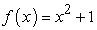and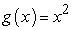, and calculated two integrals between x=1 and x=2 to obtain 10/3 for f and 7/3 for g. Subsequently, the interview progressed as shown below.

R: Can you provide a geometric or numerical example?

P: (Draws the two curves ofand) Like this?

R: Can you do this in graph form without an equation?

P: How do I draw graphs without equations?

R: OK, can you draw graphs represent the definite integral?

P: No, I do not know how to do it.

Drawing graphs based on the two functions provided, Porter was unable to think using graphical representations without algebraic formulae. Therefore, Porter’s thinking style tended to analytic but not visual, he do not understand algebra and geometry as alternative languages, represent and interpret problem graphically, and understand mathematical transformations visually. Porter used visual representations are memory image, and the graphical representations in his memory as standard graphics. An image of a standard figure may cause inflexible thinking which prevents the construction of a non-standard diagram.

Another student, John, was also categorized into the non-visual level. In Task 5, John defined the step function and then established two integrals ([–3, 0] and [0, 3]). When he was asked:

R: Why do you separate the integral into these two integrals?

J: Because of absolute value, one integral represents the value to the left of 0, and the other represents the one to the right of 0.

R: Can you draw the graph of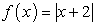?

J: (Drawing the graph correctly) Is it right?

R: Good, according to the graph, can you examine the integrals that are right or not?

J: (He think about 25 seconds) Oh, I know, the interval of integrals should between [–3, -2] and between [-2, 3].

R: Good, complete this problem.

J: (After 87 seconds) It is 13.

R: Good, can you solve this problem by the graph that you have drawn?

J: (He think about 15 seconds) I can not solve this problem by the graph.

R: OK, can you evaluate the sum of the areas of the two triangles?

J: Of course, 25/2 plus 1/2 equal to 13.

R: Good, can you tell me what the relationship between the area of triangles and the integral?

J: I know the integral is the area, and the results are the same for this problem, but this problem is to evaluate the integral…. Do you mean that I can evaluate the area instead of the integration?

R: Why not? Just you have said that the integral is the area.

J: But…

R: Can you tell me what do you think about the integral is the area?

J: I do not know exactly, I just remembered what the teacher said.

The students at the non-visual level generally used a single representation, and symbolic representation was used to solve all types of problems. This indicates that students consider symbolic representation as a support tool. Additionally, students in this group were inclined to rely on analytical thinking instead of visual thinking. This leads to they tend to be cognitively fixed on standard figures and procedures instead of recognizing the advantages of visualizing the tasks. Presmeg  showed that, visualization could be a hindrance for solving a mathematical problem, especially when a mental image of a specific subject controls the student’s thinking. In this group students’ cases the mental image of standard figure has dominated their thinking when trying to draw a figure to solve problems.

4.2. Visual Thinking of Definite Integral at the LV Level

The next level of visual thinking of the concept of definite integral regarding the existence of cognitive links and awareness of these links is the local-vision level. We categorized nine students into this group. These students understood the relationships between representation systems and could change or transfer the representations in some of the representation systems. However, these students had difficulty coordinating these relationships.

Helen was one of the students in this group. She could use correct symbolic representations to perform mathematical thinking and could manipulate the area using graphical representations according to the changes in integral symbols in Tasks 1, 4, and 5. Consider Task 1 for example, Helen assumed that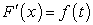, then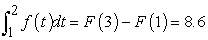. Consequently,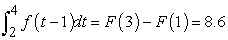. Figure 2 shows Helen’s graphical representation. She knows that because the two integrals represent the same area, so the two integrals have the same values.

However, for Task 3, she says that the proposition is false and gives graphic representations (Figure 3) but fails to make suitable justifications.

R: Can you explain what you think of this task?

H: The area enclosed by f, x = a, x = b, and the x axis is greater than the area enclosed by g, but the function value of f is smaller than g.

R: But the question involves the integral of f being greater than that of g.

H: The integral value is the area; therefore, a greater integral means a greater area.

R: Does this has any relevance to the area being above or below the x axis?

H: It is irrelevant to the area being above or below the x axis.

Similar to Helen, although the students in this group could convert symbolic representations and graphical representations, they believed that integral value was the same as area. Although they understood the relationship between the area above the x axis and the integral, they did not understand the relationship between the area below the x axis and the integral. For example, Mary calculated that the sum of the areas of the two triangles and one trapezoidal in Task 2 (Figure 4) equaled an integral value of 39/8, and that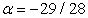; however, she did not realize that the value of was greater than -1 in the graph.

The interview results show that students in this group have begun to coordinate the representation systems of definite integrals. These students can perform representation transformations in separate representation systems. Students in this group differ from those at the non-visual level in that they have understood algebra and geometry as alternative languages for the concept of definite integral, and developed visual methods to better “see” mathematical concepts and problems. Students in this group used visual representations are induced image, they induced the visual images mainly from the analytic thinking. Although their visual thinking inclines toward local not global thinking, this restricted visualization actually hinders their solving of the tasks. Additionally, their chosen visualization only reflects one aspect of the integral concept, which has a number of significant consequences on their ability to solve the other tasks. According to Zimmerman , an important component of visual thinking is the ability to recognize that an answer obtained algebraically is false based on geometric grounds; the interviews show that this component is lacking in the minds of many students.

4.3. Visual Thinking of Definite Integral at the GV Level

Two students were categorized into this group. These students could recognize the relationships among representation systems and convert representations between representation systems. In Tasks 1, 4, and 5, Keven used correct symbolic representations to perform mathematical thinking. He also manipulated the area using graphical representations according to the changes in integral symbols. Consider Task 4 for example, Keven actually employed three methods to solve the problem. The first method was the standard algorithm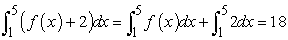; the second method was the mean value theorem for integral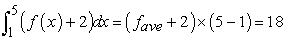; and the third method was graphical representation (Figure 5). He knows how to draw the graph of f(x)+2, and understands the relationship between the integrals and areas.

For Task 3, Keven stated that the proposition was false and provided graphical representations (Figure 6). Subsequently, the interview progressed as shown below.

R: If f(x) is greater than g(x), would the integral of f(x) be greater than the integral of g(x)?

K: Yes.

R: Why?

K: If f(x) is greater than g(x), the difference of f(x) minus g(x) would be greater than 0 and a positive value. The integral of f(x) minus g(x) would be a positive value; therefore, the integral of f(x) would be greater than the integral of g(x).

R: Why do you think Task 3 is incorrect?

K: The situation in Task 3 is opposite to that of your question. The integral values of the function in the interval [a, b] are greater, and the values of the function in the interval [a, b] are not necessarily greater than that of g(x).

K: In this graph I drew, the area below f is greater than the area below g in the interval [a, b]; therefore, the integral of f in [a, b] is greater than the integral of g in [a, b]. However, the function value of f in the interval [a, c] is smaller than the function value of g in the interval [a, c]. Therefore, the description in this task is incorrect.

Unlike students at the non-visual level who can only apply symbolic representation thinking, Keven could employ graphical representation as a thinking tool. Additionally, Keven clearly understood that the area above the x axis was the integral value, and he understood the relationship between the area under the x axis and integral (Task 2, Figure 7).

Keven clearly extract specific information from diagrams, represent and interpret problem (or concept) graphically, and understand mathematical transformations visually. The most significant differences between this group and students in the other groups were that they had the ability to perform representation treatments in representation systems, and they could perform representation conversions among various representation systems. On the other hand, the visual images that this group students used are imagination images, some kinds of spontaneous, self-construct, non-structural, a new type of organization, to integrate past and current experience in the creation of things. Imagination images different from memory images to students is, imagination images are not a real existence in the past, but through the creative process students generated. The added accuracy in this group students’ drawings could be characterized as “introducing suitable notation” which was Polya’s recommendation when using visual representations . For Keven, visualization is a powerful tool to explore mathematical problems and to ascribe meaning to the concept of definite integral and the relationship between them. Additionally, visualization reduces the complexity when considering a significant amount of information.

### 5. Conclusion

In this study, we recruited first-year calculus students at universities as the research participants to investigate visual thinking of the definite integral concept. Visual thinking involves the capacity to make connections between both mathematical objects and concepts and mathematics and the physical world. The data analysis results show that the main obstacles preventing students from freely shifting within the representation system for the concept of definite integrals were that they did not have the ability involves visualizing the abstracted relationships and non-figural information into visual representations and imagery. The development of visualization ability, which may influence the relationship between graphical representations and the other representations, increases the performance of solving definite integral problems.

The students with non- visualization ability the visual images used are memory image, the students with low visualization ability use of algebraic representations in high percentages, or use of graphical representations are induced from analytic thinking lead to the difficulties of problem solving. Possible reasons for this tendency include lecturers’ reliance of a single representation in their teaching, lecturers’ reluctance to show examples that enable the use of multiple representations leads to students’ lack of knowledge of alternative definitions. The students with high visualization ability use of graphical representations in high percentages along with algebraic representations and linking these representations lead to the success of problem solving. Aspinwall, Shaw and Presmeg  quote MacFarlane Smith as saying that “gifted individuals have their own internal ‘blackboards’ and can visualize complicated structures without being aware that they are doing so”. Although not necessarily gifted, the idea of internal blackboard seems to apply to Keven.

Moreover, the students are advised to develop their visualization abilities to improve their abilities of graphical interpretations. The development of the visual thinking ability, which is needed for the success in teaching mathematics and geometry is helpful for benefiting from different representations in solving definite integral problems.

From a didactic perspective, the introduction of graphics that illustrate a specific case and a counter-example may focus attention on the key aspects of the representational relationship and render the graphic an integral part of the concept of definite integrals. However, adopting a specific method of teaching visual thinking raises the following questions: What conversion processes are involved in moving among various mathematical representations, including those of a visual nature? How can visual, mixed, and non-visual methods be combined in class to improve the visual thinking of students?

### Acknowledgement

  Arcavi, A. “The role of visual representations in the learning of mathematics.” Educational Studies in Mathematics, 52(3). 215-241. March. 2003.In article  Aspinwall, L., and Shaw, K. “Representations in calculus: Two contrasting cases.” Mathematics Teacher, 95. 434-440. September. 2002.In article  Aspinwall, L., Shaw, K. and Presmeg, N. “Uncontrollable mental imagery: Graphical connections between a function and its derivative.” Educational Studies in Mathematics, 33. 301-317. September. 1997.In article  Berry, J., and Nyman, M. “Promoting students’ graphical understanding of the calculus.” Journal of Mathematical Behavior, 22(4). 479-495. October. 2003.In article  Dreyfus, T. “On the status of visual reasoning in mathematics and mathematics education.” In Proceedings of the Fifteenth Annual Meeting of the International Group for the Psychology of Mathematics Education, Assisi, Italy, 33-48. 1991.In article  Duval, R. “A cognitive analysis of problems of comprehension in a learning of mathematics.” Educational Studies in Mathematics, 61(1). 103-131. February. 2006.In article  Eisenberg, T., and Dreyfus, T. Visualization in Teaching and Learning Mathematics, Mathematical Association of America, Washington D.C., 1991, 25-37.In article  Goldin, G. “Representational systems, learning, and problem solving in mathematics.” Journal of mathematical behavior, 17 (2). 137-165. February. 1998.In article  Goldin, G. Handbook of Research Design in Mathematics and Science Education, Erlbaum, Mahwah, New Jersey, 2000, 517-545.In article  Haciomeroglu, E. S., Aspinwall, L. and Presmeg, N.C. “Contrasting Cases of Calculus Students' Understanding of Derivative Graphs.” Mathematical Thinking and Learning, 12(2). 152-176. March. 2009.In article  Hiebert, J., and Carpenter, T. P. Handbook of research on mathematics teaching and learning, National Council of Teachers of Mathematics, Reston, VA, 1992, 65-97.In article  Hitt, F. “Working Group on Representations and Mathematics Visualization. In Proceedings of the Twentieth Annual Meeting of the North American Chapter of the International Group for the Psychology of Mathematics Education, North Carolina. USA. Columbus, 1-7. November. 1998.In article  Hughes-Hallett, D., McCallum, W. G., Gleason, A. M., Pasquale, A., Flath, D. E., Quinney, D., Lock, P. F., Raskind, W., Gordon, S. P., Rhea, K., Lomen, D. O., Tecosky-Feldman, J., Lovelock, D., Thrash, J. B., Osgood, B. G., and Tucker, T. W. (2002). Calculus: Single Variable. MA: John Wiley & Sons, Inc, Danvers, 2002.In article  Kaput, J. J. Problems of representation in teaching and learning mathematics, Erlbaum, Hillsdale, New Jersey, 1987, 19-26.In article  Mahir, N. “Conceptual and procedural performance of undergraduate students in integration.” International Journal of Mathematical Education in Science and Technology, 40(2). 201–211. January. 2009.In article  Nemirovsky, R., and Noble, T. “On Mathematical Visualisation and the Place Where We Live.” Educational Studies in Mathematics 33(2). 595-610. July. 1997.In article  Noss, R., Healy, L. and Hoyles, C. “The Construction of Mathematical Meanings: Connecting the Visual with the Symbolic.” Educational Studies in Mathematics, 33(2). 203-33. July. 1997.In article  Pólya, G. How to solve it, Princeton University Press, Princeton, New Jersey, 1945.In article  Presmeg, N.C. Handbook of Research on the Psychology of Mathematics Education: Past, Present and Future. Sense Publishers, Rotterdam, 2006, 205-235.In article  Sealey, V. “Definite integrals, Riemann sums, and area under a curve: what is necessary and sufficient?” In Proceedings of the 28th annual meeting of the North American Chapter of the International Group for the Psychology of Mathematics Education, Mérida, México: Universidad Pedagógica Nacional, 46-53. November. 2006.In article  Stylianou, D.A., and Silver, E.A. “The role of visual representations in advanced mathematical problem solving: An examination of expert-novice similarities and differences.” Mathematical Thinking and Learning, 6(4). 353-387. November. 2004.In article  von Glasersfeld, E. Problems of representation in the teaching and learning of mathematics, Lawrence Erlbaum Associates, Inc, New Jersey, 1987, 215-225.In article  Yoon, C., Thomas, M. O. J., and Dreyfus, T. “Gestures and virtual space.” In Proceedings of the 33rd Conference of the International Group for the Psychology of Mathematics Education, Thessaloniki, Greece, 409-416. 2009.In article PubMed  Zazkis, R., Dubinsky, E. and Dautermann, J. “Coordinating visual and analytic strategies: a study of students’ understanding.” Journal for Research in Mathematics Education, 27(4). 435-437. July. 1996.In article  Zimmermann, W. Visualization in teaching and learning mathematics, Mathematical Association of America, Washington, D.C., 1991, 127-138.In article  Zimmermann, W., and Cunningham, S. Visualization in Teaching and Learning Mathematics, Mathematical Association of America, Washington, D.C., 1991, 1-8.In article PubMed